Home    |    Teacher    |    Parents    |    Glossary    |    About UsYou can visualize positive and negative integers using the number line.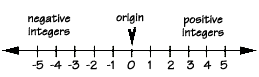It's important to understand the number line because it shows you that every number has an opposite. The famous German mathematician Leopold Kronecker once said: "God made the positive integers; everything else is the work of man." Why, then, did we confuse things with negative numbers? As it turns out, there are many, many everyday problems where negative numbers are useful. For example, we can both gain and lose weight.

The temperature can rise or fall. Locations on the earth can be above sea level, or below sea level.

An integer is a whole number that can be either greater than 0, called positive, or less than 0, called negative. Zero is neither positive nor negative. Two integers that are the same distance from the origin in opposite directions are called opposites.

The arrows on each end of the number line show us that the line stretches to infinity in both the negative and positive direction. We don't have to include a positive sign (+) when we write positive numbers. However, we do have to include the negative sign (-) when we write negative numbers. Zero is called the origin, and it's neither negative nor positive.

For every positive integer, there's a negative integer an equal distance from the origin. Two integers that lie the same distance from the origin in opposite directions are called opposites. For example, "negative 5" is the opposite of "positive 5."

Every number on the number line also has an absolute value, which simply means how far that number is from zero. The symbol for absolute value is two vertical lines. Since opposites are the same distance from the origin, they have the same absolute value. For example, the absolute value of "negative 10" is ten, and the absolute value of "positive 10" is also 10. The absolute value of zero is zero.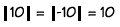Examples

• 2 is less than 5 because … 2 lies to the left of 5
• -1 is greater than -3 because … -1 lies to the right of -3
• -4 is less than 1 because … -4 lies to the left of 1
• 6 is greater than -2 because … 6 lies to the right of -2

Which is greater: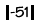or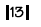?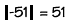because the absolute value of a negative number is its opposite.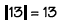because the absolute value of a positive number is itself.

51 is greater than 13

Find: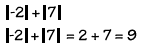Homework Help | Pre-Algebra | NumbersEmail this page to a friendSearch·  Place value·  Decimal numbers·  Estimating and     rounding·  Adding / subtracting     decimals·  Multiplying decimals·  Dividing decimals·  Percent·  Exponents·  Square roots·  Signed integers·  Adding and     subtracting integers·  Multiplying and     dividing integers·  Properties of integersFirst Glance In Depth Examples WorkoutSigned Integers